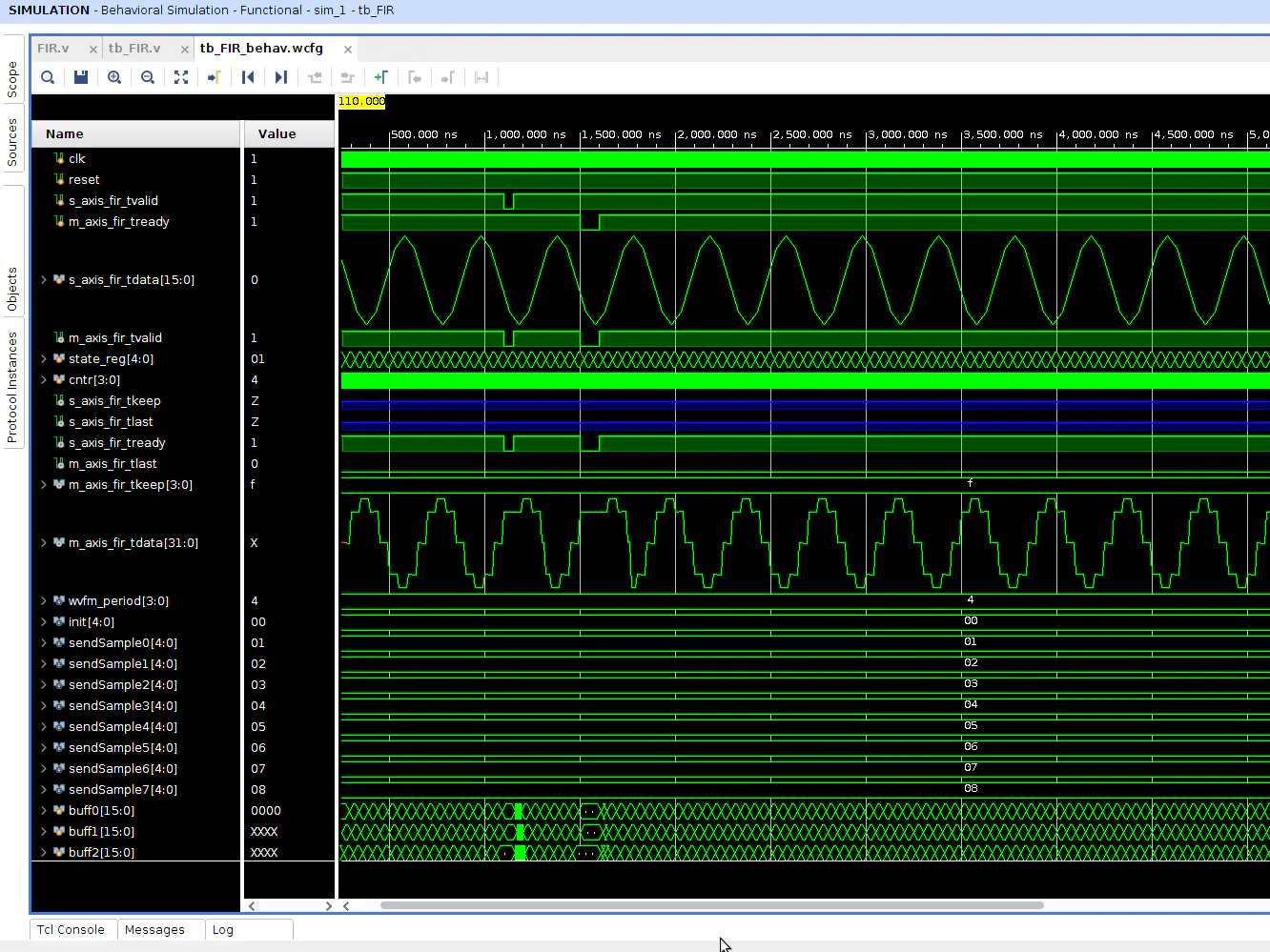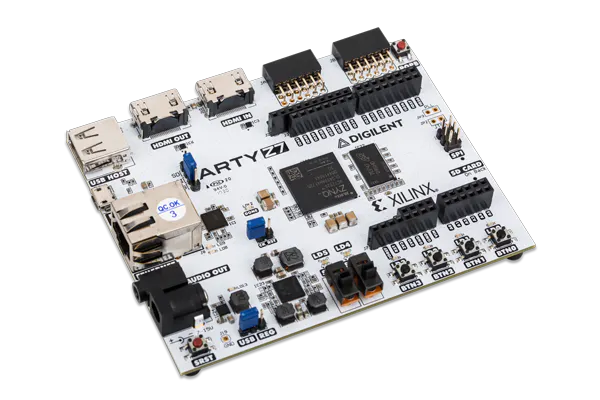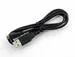# DSP for FPGA: Rewriting FIR Logic to Meet Timing

Continuing with my simple FIR filter Verilog module, this project walks through how to rewrite HDL logic when setup timing violations occur.

IntermediateFull instructions provided2 hours2,494## Things used in this project

### Hardware componentsDigilent Arty Z7-20
×1USB-A to Micro-USB Cable
×1

### Software apps and online services## Code

### FIR.v

Verilog
````timescale 1ns / 1ps

module FIR(
input clk,
input reset,
input signed [15:0] s_axis_fir_tdata,
input [3:0] s_axis_fir_tkeep,
input s_axis_fir_tlast,
input s_axis_fir_tvalid,
output reg m_axis_fir_tvalid,
output reg m_axis_fir_tlast,
output reg [3:0] m_axis_fir_tkeep,
output reg signed [31:0] m_axis_fir_tdata
);

/* This loop controls tkeep signal on AXI Stream interface */
always @ (posedge clk)
begin
m_axis_fir_tkeep <= 4'hf;
end

/* This loop controls tlast signal on AXI Stream interface */
always @ (posedge clk)
begin
if (s_axis_fir_tlast == 1'b1)
begin
m_axis_fir_tlast <= 1'b1;
end
else
begin
m_axis_fir_tlast <= 1'b0;
end
end

// 15-tap FIR
reg enable_fir;
reg signed [15:0] buff0, buff1, buff2, buff3, buff4, buff5, buff6, buff7, buff8, buff9, buff10, buff11, buff12, buff13, buff14;
wire signed [15:0] tap0, tap1, tap2, tap3, tap4, tap5, tap6, tap7, tap8, tap9, tap10, tap11, tap12, tap13, tap14;
reg signed [31:0] acc0, acc1, acc2, acc3, acc4, acc5, acc6, acc7, acc8, acc9, acc10, acc11, acc12, acc13, acc14;

/* Taps for LPF running @ 1MSps */
assign tap0 = 16'hFC9C;  // twos(-0.0265 * 32768) = 0xFC9C
assign tap1 = 16'h0000;  // 0
assign tap2 = 16'h05A5;  // 0.0441 * 32768 = 1445.0688 = 1445 = 0x05A5
assign tap3 = 16'h0000;  // 0
assign tap4 = 16'hF40C;  // twos(-0.0934 * 32768) = 0xF40C
assign tap5 = 16'h0000;  // 0
assign tap6 = 16'h282D;  // 0.3139 * 32768 = 10285.8752 = 10285 = 0x282D
assign tap7 = 16'h4000;  // 0.5000 * 32768 = 16384 = 0x4000
assign tap8 = 16'h282D;  // 0.3139 * 32768 = 10285.8752 = 10285 = 0x282D
assign tap9 = 16'h0000;  // 0
assign tap10 = 16'hF40C; // twos(-0.0934 * 32768) = 0xF40C
assign tap11 = 16'h0000; // 0
assign tap12 = 16'h05A5; // 0.0441 * 32768 = 1445.0688 = 1445 = 0x05A5
assign tap13 = 16'h0000; // 0
assign tap14 = 16'hFC9C; // twos(-0.0265 * 32768) = 0xFC9C

/* This loop controls tready & tvalid signals on AXI Stream interface */
always @ (posedge clk)
begin
if(reset == 1'b0 || m_axis_fir_tready == 1'b0 || s_axis_fir_tvalid == 1'b0)
begin
enable_fir <= 1'b0;
m_axis_fir_tvalid <= 1'b0;
end
else
begin
enable_fir <= 1'b1;
m_axis_fir_tvalid <= 1'b1;
end
end

reg [3:0] cnt;
reg signed [31:0] acc01, acc012, acc23, acc34, acc45, acc56, acc67, acc78, acc89, acc910, acc1011, acc1112, acc1213;

/* Circular buffer w/ Multiply & Accumulate stages of FIR */
always @ (posedge clk or posedge reset)
begin
if (reset == 1'b0)
begin
m_axis_fir_tdata <= 32'd0;
end
else if (enable_fir == 1'b1)
begin
buff0 <= s_axis_fir_tdata;
acc0 <= tap0 * buff0;

buff1 <= buff0;
acc1 <= tap1 * buff1;
acc01 <= acc0 + acc1;

buff2 <= buff1;
acc2 <= tap2 * buff2;
acc012 <= acc01 + acc2;

buff3 <= buff2;
acc3 <= tap3 * buff3;
acc23 <= acc012 + acc3;

buff4 <= buff3;
acc4 <= tap4 * buff4;
acc34 <= acc23 + acc4;

buff5 <= buff4;
acc5 <= tap5 * buff5;
acc45 <= acc34 + acc5;

buff6 <= buff5;
acc6 <= tap6 * buff6;
acc56 <= acc45 + acc6;

buff7 <= buff6;
acc7 <= tap7 * buff7;
acc67 <= acc56 + acc7;

buff8 <= buff7;
acc8 <= tap8 * buff8;
acc78 <= acc67 + acc8;

buff9 <= buff8;
acc9 <= tap9 * buff9;
acc89 <= acc78 + acc9;

buff10 <= buff9;
acc10 <= tap10 * buff10;
acc910 <= acc89 + acc10;

buff11 <= buff10;
acc11 <= tap11 * buff11;
acc1011 <= acc910 + acc11;

buff12 <= buff11;
acc12 <= tap12 * buff12;
acc1112 <= acc1011 + acc12;

buff13 <= buff12;
acc13 <= tap13 * buff13;
acc1213 <= acc1112 + acc13;

buff14 <= buff13;
acc14 <= tap14 * buff14;
m_axis_fir_tdata <= acc1213 + acc14;

end
end

endmodule
```

## Credits

### Whitney Knitter

78 projects • 891 followers
Working as a full-time SDR/FPGA engineer, but making time for the fun projects at home.